Study Guide

# Sequences

## Sequences

Sequence is a big word. It can sound scary, like an impending thunderstorm heading your direction, approaching with thunderous laughs. Sometimes we could swear we saw a set of evil eyes looking ominously at us from the clouds.

Don't worry. A sequence is just a list of numbers. For example, pick an integer and right it down. Now pick two more and write them down behind the first. Separate each number with a comma. You just made a sequence. Nothing scary about that, right? The individual numbers you just wrote are called terms. The sequence you just made up should have three terms. Because it has three terms–the list of numbers ends–it's finite.

Let's make it a little more complicated. Get out your writing hand of non-stop, legible scribble and your infinitely long #2 pencil. No, this is not a never-ending standardized exam. Start writing down numbers, separated by a comma, and never stop. That sequence is infinite.

Be Careful: In math, sequences and series are not the same thing.

We can write down a finite sequence by writing down its terms with commas in between. If a sequence is infinite, we don't need a special hand or #2 pencil. Instead, we write down some of its terms, and then we write some dots (...) to indicate that the sequence continues forever in the same pattern.

### Sample Problem

The following are finite sequences:

1, 2, 3

1, 1, 1, 1, 1, 1, 1, 1

The following are infinite sequences:

1, 1, 1,...

1, 2, 3,....

While we might only encounter infinite sequences in calculus class, nothing about the definition says sequences have to be infinite. Finite sequences are okay, too.

• ### Defining Sequences and Evaluating Terms

Let's imagine, instead of numbers, we have a sequence of animals. The sequence begins with three animals: green tap dancing elephant, purple singing cockatoo, and orange quarterback tiger. We could refer to the first term as green tap dancing elephant, the second as the purple singing cockatoo, and so on. But we'll just become confused in our circus of exotic animal talent.

Or, we could just give each term of the sequence a short label.

We call the first term of a sequence a1 (the elephant in our circus), the second term a2 (the cockatoo), and so on:

a1a2, a3, ..., an, ...

The nth term, an, is called the general term of a sequence.

Sometimes it's useful to think of a sequence as starting with a "zeroth" term instead of a "first" term. Then we would think of the sequence as

a0, a1a2, a3, ..., an, ...

It sounds like we're just being difficult, defining a term by a number that means none. We aren't. We'll tell you why later. For now, assume sequences start with n = 1 unless told otherwise.

With sequences, we like to know how to get there from here. To describe or define a sequence we need to know how to find the general term. It's like knowing how to get from your couch, where you were reading up on Greek mythology for giggles, to the kitchen to fetch a slice of watermelon.

This description may or may not involve a mathematical formula. Some sequences can't be described by a nice tidy formula.

### Sample Problem

Define a sequence by

an = high temperature at Shmoop HQ, on the nth day after January 1, 2010.

This is a perfectly reasonable definition. However, as far as anyone knows, there is no mathematical formula that can produce the nth term. Despite our extensive knowledge of rain dancing, we can't predict the weather that accurately.

Even if it's usually 75 and sunny at Shmoop HQ.

• ### Patterns

Evaluating terms of a sequence, given the formula, isn't so bad. Going the other way around is a little trickier. It can be a bit like juggling buzzing chainsaws while riding a unicycle and chewing gum.

Okay, it's not that hard. Given the terms, how do you figure out the formula?

### Sample Problem

Write down the formula for the general term in the sequence

2,4,6,8,10,...

starting with n = 1.

Let's make a table so we can see the relationship between n and an.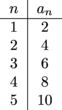The second number in each row is obtained by doubling the first number.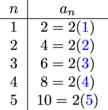The formula for the general term is

an = 2n.

You should be very familiar with the following common sequences and the definitions of their general terms.

• The sequence of natural numbers an = n:
1, 2, 3, 4,...

• The sequence of squares an = n2:
1, 4, 9, 16, 25,...

• The sequence of cubes an = n3:
1, 8, 27, 64,...

• The sequence of even numbers an = 2n:
2, 4, 6, 8,...

• The sequence of odd numbers an = 2n – 1:
1, 3, 5, 7,...
We can also write this sequence as an = 2n + 1 if we start with n = 0 instead of n = 1.

• The sequence of powers of 2 where an = 2n:
2, 4, 8, 16, 32,...

Most people don't want to reinvent the wheel, and mathematicians are no exception. Many sequences are built by making slight adjustments to more familiar sequences. Adjustments might include adding, subtracting, or multiplying by a constant.

In most of the sequences we've looked at so far, n only shows up once. There's only one occurrence of n in the formula an = n2 or an = 2n.

The most complicated general term we've seen is an = n2 + n.

When terms get more complicated, finding a formula for the nth term can feel like trying to solve a Rubik's cube. Just like the Rubik's cube, we have to look at individual parts to figure out the general formula.

Here's a useful trick. When you get to Taylor series, you'll need to be comfortable with sequences whose terms have alternating signs.

### Sample Problem

Find a formula for the general term of the sequence

-1, 1, -1, 1,...

starting at n = 1.

If we raise (-1) to an odd power, we get -1. If we raise (-1) to an even power, we get + 1. We could think of the sequence

-1, 1, -1, 1,...

as

(-1)1, (-1)2, (-1)3, (-1)4, ...

Then the formula for the general term is

an = (-1)n.

### Sample Problem

Find a formula for the general term of the sequence

1, -1, 1, -1,...

starting at n = 1.

There are (at least) two answers. We can think of this sequence as

(-1)2,(-1)3,(-1)4,(-1)5,...

in which case the general term is

an = (-1)n + 1.

Or we can think of the sequence as

(-1)0,(-1)1,(-1)2,(-1)3,...

in which case the general term is

an = (-1)n – 1.

Either formula is right.

If the terms of a sequence have alternating signs, the formula for the general term will have a factor of (-1) raised to some power.

Using this trick is simple. To find the formula for such a sequence, first ignore the alternating signs and find the formula you would have if all terms were positive. Then multiply by (-1)n or (-1)n + 1 to account for the signs.

Next, we will teach you how to amaze your friends by levitating six inches off the floor.

• ### Sequences Can Start at n = 0

Remember the Count from Sesame Street? Now a little bit older and with a few gray hairs, he needs a good pick-me-up in the morning, "One. One large coffee. Ha.Ha.Ha." Why would we begin counting sequences from zero? The Count never started counting from 0.

Sometimes it's easier. Computer programmers start from 0 all the time, and a sequence can start from the zeroth term, n = 0, too. It doesn't matter. We have the freedom to start from whatever number n we want. We can be rebels and begin from n = 194.

We need to keep mind, though, that the formula for the general term of a sequence will vary depending on the starting value of n. While we can start a sequence at any value of n we like, n = 0 and n = 1 are the most common starting values. They are the easiest to work with. And they are the two neatest numbers we have.

Here are some variations on the common sequences that show up when we start a sequence at n = 0:

• The sequence of whole numbers numbers an = n:
0, 1, 2, 3, 4,...

• The sequence of even numbers an = 2n:
0, 2, 4, 6, 8,...

• The sequence of odd numbers an = 2n + 1:
1, 3, 5, 7,...

• The sequence of powers of 2 where an = 2n:
1, 2, 4, 8, 16, 32,...

Formulas for alternating sequences of ±1 also get affected by the starting value of n. When we started at n = 1, the sequence

-1, 1, -1, 1,...

was given by

an = (-1)n.

If instead we start at n = 0, this sequence is given by

an = (-1)n + 1.

To make sure you have the correct exponent on your (-1) factor, evaluate the first term of the sequence and make sure it has the correct sign.

If you're asked to find the formula for the general term of a sequence and not given the starting value of n, you should choose n = 0 or n = 1. It can seem a bit like choosing between plain or peanut M&Ms. If you are in the mood for a chocolatey start and a peanutty finish, start from n = 0. And sometimes, one starting value may seem more convenient than the other.

### Sample Problem

Find a formula for the general term of the sequence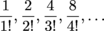If we look at the numerators first, we see the sequence

1, 2, 4, 8,...

These are the powers of 2 starting at n = 0. Take n = 0 as the starting point and look at the denominators: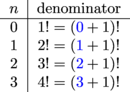The denominator is given by (n + 1)!. The sequence is given by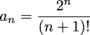, starting at n = 0.

If we look at the denominators first, we see the sequence

1!, 2!, 3!, 4!, ...

These are the factorials, starting at n = 1. Take n = 1 as the starting point and look at the numerators: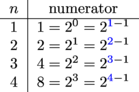The numerator is 2n – 1. The sequence is given by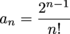, starting at n = 1.

In the example above, we got two possible formulas depending on the starting value of n: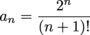or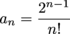In the first formula, the exponent (n) is simple and the factorial ((n + 1)!) is complicated. In the second formula, the exponent (n – 1) is complicated and the factorial (n!) is simple. In the end, it doesn't matter which formula you pick. You have the power--pun intended--to begin wherever you like.

Sometimes one formula and starting value will be preferable over the other. To write the even numbers

0, 2, 4, 6, 8,...

we want to start with n = 0 so the general term can be

an = 2n. If we started with n = 1 the general term would be

an = 2n – 2, which is more complicated than necessary.

In cases like this, use the simple answer. Picking n so you have a simpler formula will save you time. Maybe you can use that extra time to stick your younger brother's favorite shoes to the ceiling with super glue.